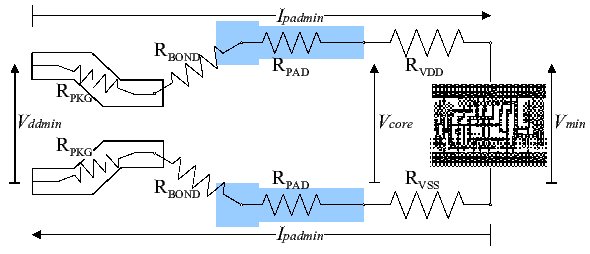The following terminology is used for power, voltage and current at nominal and worst-case conditions.

 Pnom Pmin Chip power consumption Vdd Vddmin External voltage Inom Imin Total current supplied to chip core Ipad Ipadmin Average current through each core supply pad Vcore Minimum voltage at edge of core Vmin Minimum voltage at centre of core

The values of Pnom, Vdd, Vddmin and Vmin are part of the chip spec. The other values are related by the following expressions, where Npad is the number of core Vdd pads or core Vss pads.

 Pmin = Pnom × Vddmin2 Vdd2
 Inom = Pnom Vdd
 Imin = Pmin = Pnom×Vddmin = Inom × Vddmin Vddmin Vdd2 Vdd

Referring to the figure on the right, the voltage at the edge of the core Vcore is related to the external voltage Vddmin, the current flow Ipadmin through the supply pad and the resistances of the package leadframe, the bond wires and the core power supply pad.

VddPicture showing the relationship between Vcore, the external voltage and the package, bond and core supply pad resistances.

We can express Ipadmin as a function of the IR drop and then equate this to the value on the left where it is a function of the core power. This way we have an expression that links the core power to the IR drop.

 (Vcore−Vmin) as a function of IR drop Rsup×Npad
=
 Pnom×Vddmin as a function of core power Npad×Vdd2

where Rsup=Rvdd=Rvss, taking the supply power to be symmetrical.

Rearranging gives

 Pnom = (Vcore−Vmin)×Vdd2 × 1 Vddmin Rsup